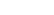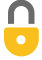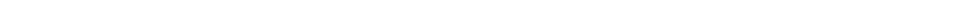Problem 30
Q

# An iron boiler of mass 180 kg contains 730 kg of water at $18 ^\circ \textrm{C}$. A heater supplies energy at the rate of 58,000 kJ/h. How long does it take for the water

1. to reach the boiling point, and
2. to all have changed to steam?A
1. $4.4 \textrm{ h}$
2. $33 \textrm{ h}$In order to watch this solution you need to have a subscription.VIDEO TRANSCRIPT

This is Giancoli Answers with Mr. Dychko. Power is energy over time. And we can solve this for t by multiplying both sides by t over p. And the left side becomes just time. And on the right hand side we have the t's cancelling and it's Q over p. So, time is energy absorbed divided by power. And in case a, the energy absorbed is the energy needed to heat the water up to its final temperature plus the energy needed to heat the iron container up to the same temperature. So, they both have the same change in temperature, both, the water and the iron boiler that it's in start with the same initial temperature and they both reach the same final temperature. So, factor out the ΔT. And you get the ΔT is times mass of the water times specific heat of water plus mass of the iron times specific heat of iron. And all this becomes Q in the time formula. So, that time or that Q energy absorbed by both, the water and iron boiler, get divided by the power. And you get the time, you have to heat it up to 100 degrees Celsius. So, the change in temperature is 100 minus 18 degrees Celsius times by the mass of water of 730 kilograms times specific heat of water, 4,186 joules per kilogram Celsius degree, plus the 180 kilograms iron that makes up the boiler times 450 joules per kilogram Celsius degree, specific heat of iron, divided by 58,000 times 10 to the 3 joules per hour, power output of the boiler. And that gives about 4.4 hours. Since we didn't convert this into joules per second or watts, we're left with units of hours in this case. And then the total time to heat the water up to 100 degrees Celsius and then also to boil it and changes its phase from liquid into gas, we're gonna have to take the time that we already know to heat it up to 100 degrees and then add to that the time needed to give it as much energy as required to change its phase from liquid to gas which is its mass times its latent heat of vaporization divided by power. So, that's 4.43476 hours plus 730 kilograms times 22.6 times 10 to the 5 joules per kilogram, latent heat of vaporization, divided by the power. And that's going to make 33 hours in total.

Giancoli Answers, including solutions and videos, is copyright © 2009-2023 Shaun Dychko, Vancouver, BC, Canada. Giancoli Answers is not affiliated with the textbook publisher. Book covers, titles, and author names appear for reference purposes only and are the property of their respective owners. Giancoli Answers is your best source for the 7th and 6th edition Giancoli physics solutions.